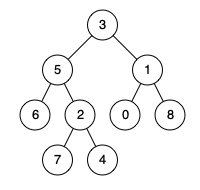# LeetCode: 236. Lowest Common Ancestor of a Binary Tree

## 题目¶

Given a binary tree, find the lowest common ancestor (LCA) of two given nodes in the tree.

According to the definition of LCA on Wikipedia : “The lowest common ancestor is defined between two nodes p and q as the lowest node in T that has both p and q as descendants (where we allow a node to be a descendant of itself).”

Example 1:```Input: root = [3,5,1,6,2,0,8,null,null,7,4], p = 5, q = 1
Output: 3
Explanation: The LCA of nodes 5 and 1 is 3.
```

Example 2:```Input: root = [3,5,1,6,2,0,8,null,null,7,4], p = 5, q = 4
Output: 5
Explanation: The LCA of nodes 5 and 4 is 5, since a node can be a descendant of itself according to the LCA definition.
```

Example 3:

```Input: root = [1,2], p = 1, q = 2
Output: 1
```

Constraints:

• The number of nodes in the tree is in the range [2, 10^5].
• -10^9 <= Node.val <= 10^9
• All Node.val are unique.
• p != q
• p and q will exist in the tree.

## 解法¶

• 如果当前 root 节点为 None ，则返回 None
• 如果当前 root 节点节点值等于 p 或 q 的值，则当前节点即为要找的 LCA，因为当前节点是 p 或 q 其中一个节点，不会有比它更近的共同祖先了。
• 在左子树中查找，假设结果为 left
• 在右子树中查找，假设结果为 right
• 如果 left 和 right 都不为 None，说明 left 和 right 刚好就是 p 和 q 这两个节点， 那么当前 root 节点即为要找的 LCA
• 否则的话，left 和 right 中哪个不为 None，哪个就是要找的 LCA

```# Definition for a binary tree node.
# class TreeNode(object):
#     def __init__(self, x):
#         self.val = x
#         self.left = None
#         self.right = None

class Solution(object):
def lowestCommonAncestor(self, root, p, q):
if root is None:
return None

if root.val == p.val or root.val == q.val:
return root

left = self.lowestCommonAncestor(root.left, p, q)
right = self.lowestCommonAncestor(root.right, p, q)

if left is not None and right is not None:
return root

if left is not None:
return left
if right is not None:
return right
```